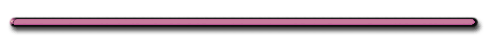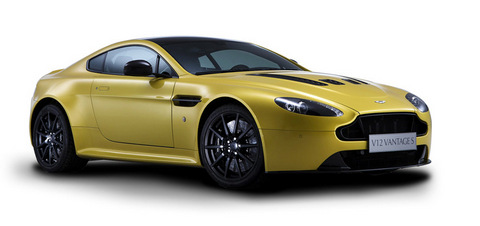Distance and Motion - FlashVideo Instruction

showmethephysics.com

Aston Martin V12 Vanquish SWhat do fancy sports cars brag about

Acceleration

C. Acceleration - time rate of change in velocity (how quickly velocity is changing)

Rank the accelerations below from the smallest to greatest?

 t(sec) t = 0 t = 1 t = 2 t = 3 t = 4 t = 5 Car A 70 m/s 72 m/s 74 m/s 76 m/s 78 m/s 80 m/s Car B 0 m/s 3 m/s 6 m/s 9 m/s 12 m/s 15 m/s Car C 90 m/s 90 m/s 90 m/s 90 m/s 90 m/s 90 m/s Car D 100 m/s 99 m/s 98 m/s 97 m/s 96 m/s 95 m/s

Car A

+2 m/s/s

Car B

+3 m/s/s

Car C

0 m/s/s

Car D

- 1 m/s/s

 t(sec) t = 0 t = 1 t = 2 t = 3 t = 4 t = 5 Car A 70 m/s 72 m/s 74 m/s 76 m/s 78 m/s 80 m/s Car B 0 m/s 3 m/s 6 m/s 9 m/s 12 m/s 15 m/s Car C 90 m/s 90 m/s 90 m/s 90 m/s 90 m/s 90 m/s Car D 100 m/s 99 m/s 98 m/s 97 m/s 96 m/s 95 m/s

2 Ways to Have
Zero Acceleration

a) Remain at Restb) Constant Velocity1. Equation

a = ∆V/∆t  = (Vf - Vi)/t

Ex 1Starting Velocity: 5m/s West

Final Velocity: 25 m/s West

Time during speed change: 5 secs

a = (Vf - Vi)/(tf - ti)

a = 20 m/s W/5 sec

 = 4 m/s West s

(multiply top and bottom by seconds)

or 4 m/s2 West

Ex 2)Starting Velocity: 20m/s West

Final Velocity:5 m/s West

Time: 5 seconds

a = (Vf - Vi)/(tf -ti)

a = -15m/s W/5 seconds =

= - 3 m/s/s West

or -3 m/s2  W

or 3 m/s2 East

Object is said to be'Accelerating Backwards'

(Because the force required to slow an object
always opposes the objects motion)